#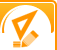Mathematics

Mathematics, considered the underlying structure of all science, is the study of properties, statistics, measurementation, and relation of quantities and sets using number and symbols. Essentially, mathematics is the science of structure, order, and relation.

All projects

• Bernoulli’s Principle

To demonstrate Bernoulli’s Principle

• Checking vs. Savings

To determine which type of account yields the most profit based on interest rate on a \$50 balance. You can take this experiment one step further to determine which bank in your area offers the best annual interest rates on the same types of accounts.

• Math and Gender

To determine if there is a difference between the overall math test scores of girls and boys and to determine if this is a direct reflection of gender related superior mathematical ability.

• Mean, Median and Range

To determine whether the mean, median and range of the height of students will differ from one grade to the next.

• Play-Doh and Volume

To determine whether Play-Doh loses volume after it’s played with or if it the amount of Play-Doh never changes.

• Refraction in Water

To demonstrate the principle of refraction of light rays in water.

• Statistics and M&M’s

To determine how probable it is that you will reach in a jar and pull out a certain color of M&M.Hide all projects# All Projects List

•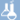Chemistry
•Biology
•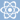Physics
•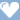Medicine & Health
•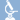Biochemistry
•Environmental science
•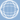Earth science
•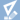Mathematics
•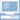Computer science